# A Physicist's Crash Course on Artificial Neural Network¶

Before I start anything, I would express my thanks to Shashank.

In :
# This cell is to prepare the notebook. Skip to the next section

# This line configures matplotlib to show figures embedded in the notebook,
# instead of opening a new window for each figure. More about that later.
# If you are using an old version of IPython, try using '%pylab inline' instead.
%matplotlib inline
import matplotlib.pyplot as plt
import numpy as np

from scipy.optimize import minimize
from scipy.special import expit

import timeit

from IPython.display import Image


## The Crash Course¶

This the theory presented in a introductory manor.

### What is a Neuron¶

What a neuron does is to response when a stimulation is given. This response could be strong or weak or even null. If I would draw a figure, of this behavior, it looks like this.

In :
Image(filename='assets/images/neuronResponse.png',width=900)

Out: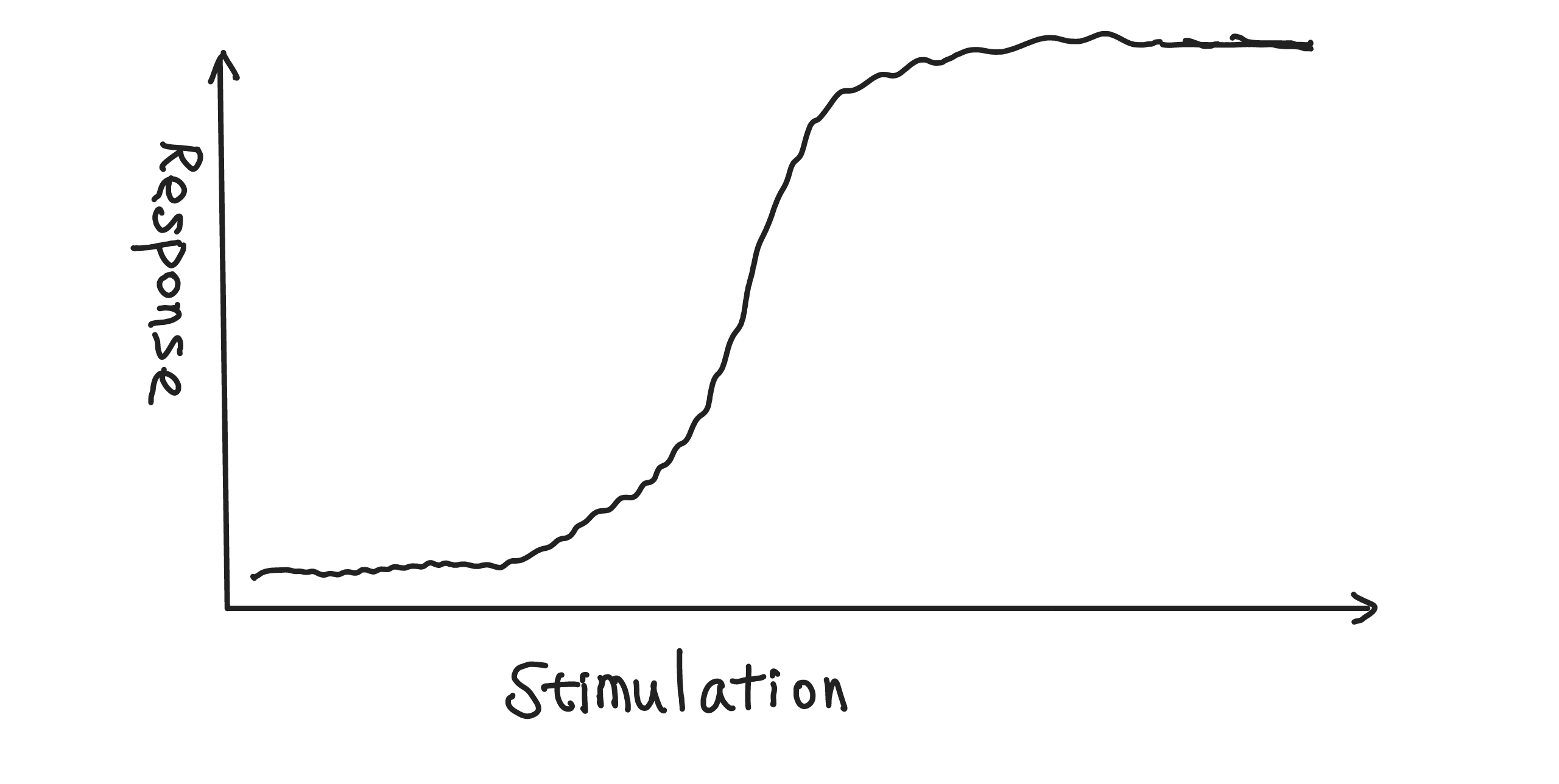However, each neuron is different from other neurons.

In :
Image(filename='assets/images/transformActivation.png',width=900)

Out: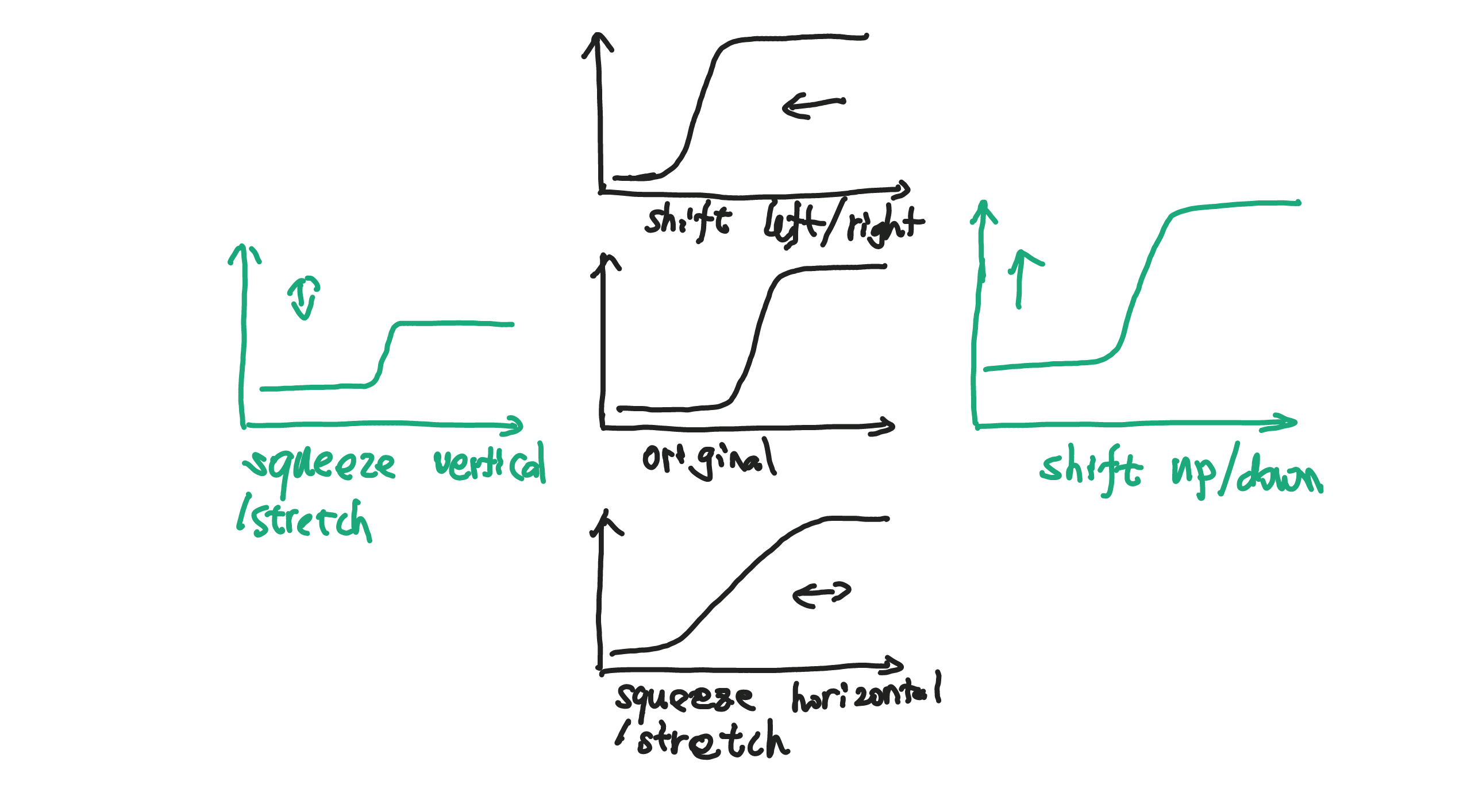### Artificial Neural Network¶

A simple network is a collection of neurons that response to stimulations, which could be the responses of other neurons.

In :
Image(filename='assets/images/neuralNetworkSimple.png',width=900)

Out: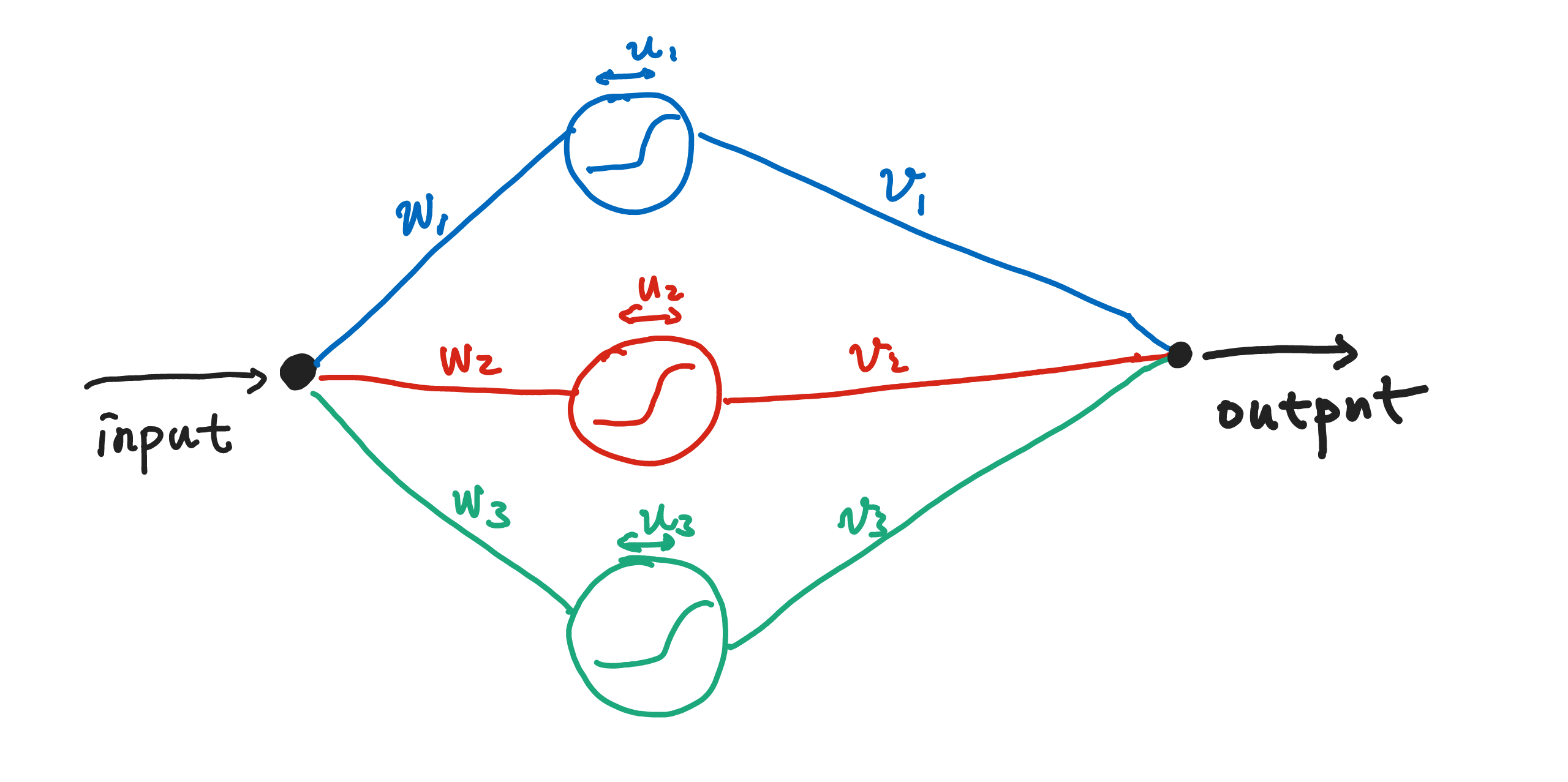A given input signal is spreaded onto three different neurons. The neurons respond to this signal sperately then summed together with different weights. In the language of math, given input $x$, output $y(x)$ is

$$y(x) = \sum_{k=1}^{3} x v_k * \text{activation}( w_k * x + u_k )$$

where $\text{activation}$ is the activation function, i.e., the response behavior of the neuron. This is a single layer structure.

A lot of different ways could be used to extend this network.

• Increase the number of neurons on one layer.
• One can extend the number of layers.
In :
Image(filename='assets/images/multilayer.png',width=900)

Out: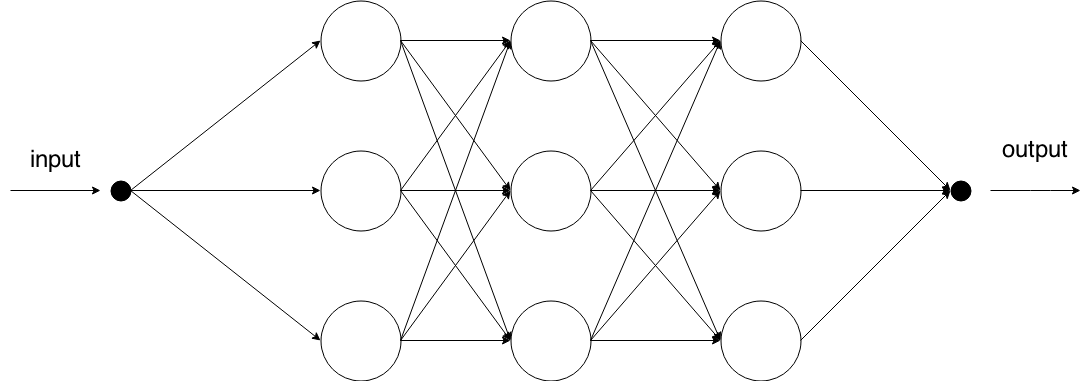• We could also include interactions between the neurons.
• Even memory can be simulated.

### How it works¶

Here is an exmaple of how the network works.

We are going to do two things.

1. Find out which temperature is hot which is cold.
2. Find out which room is habitable in terms of temperature.

The first task can be done using one neuron. If a set of parameters are properly chosen, a single neuron will finish the task.

We have a input temperature and a output that tells us which is high temperature which is low temperature. In the following example, $T_1$ is low temperature, $T_2$, $T_3$ are high temperatures.

In :
Image(filename='assets/images/1neuronClassification.png',width=900)

Out: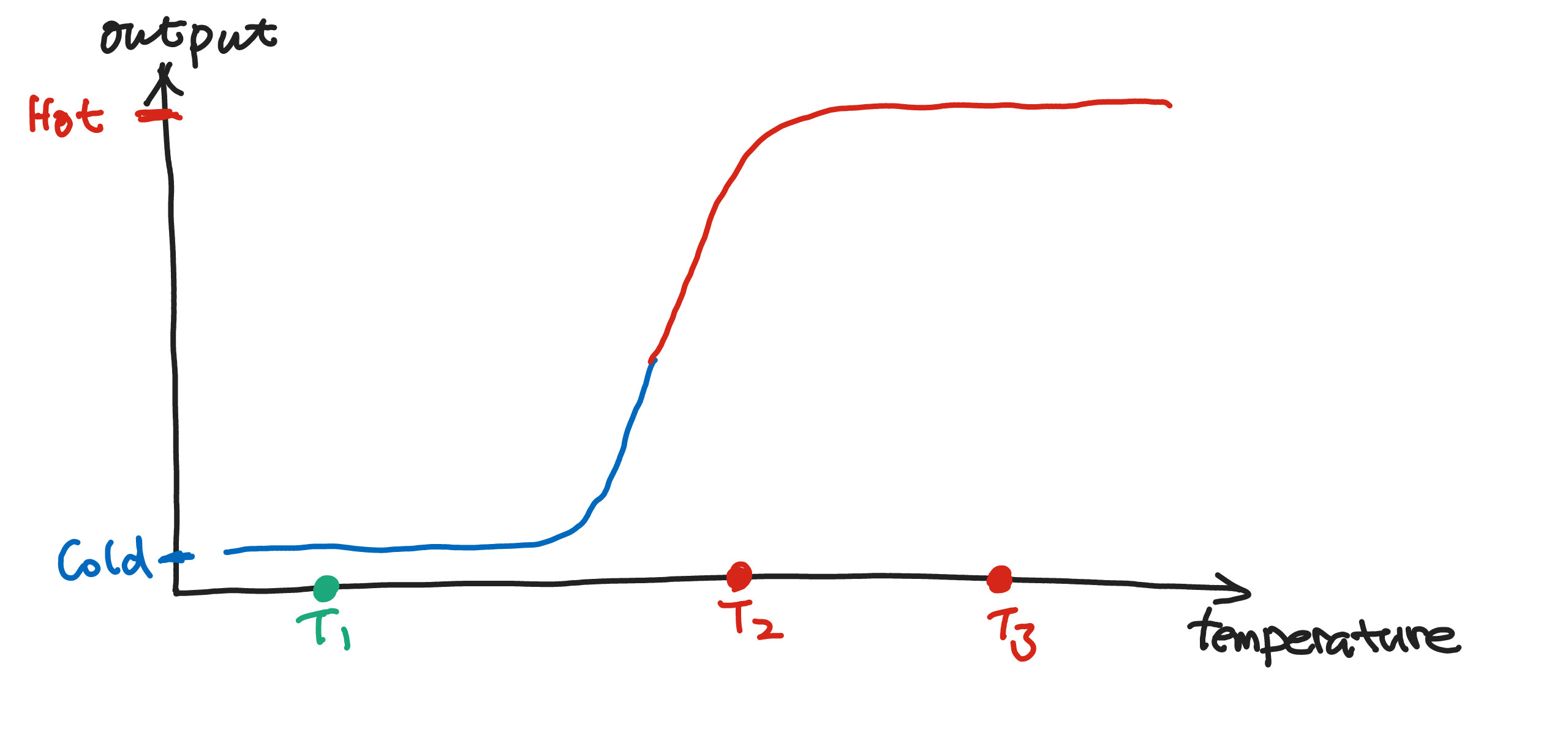Suppose we have only two neurons in the network.

In :
Image(filename='assets/images/2neuronNet.png',width=900)

Out: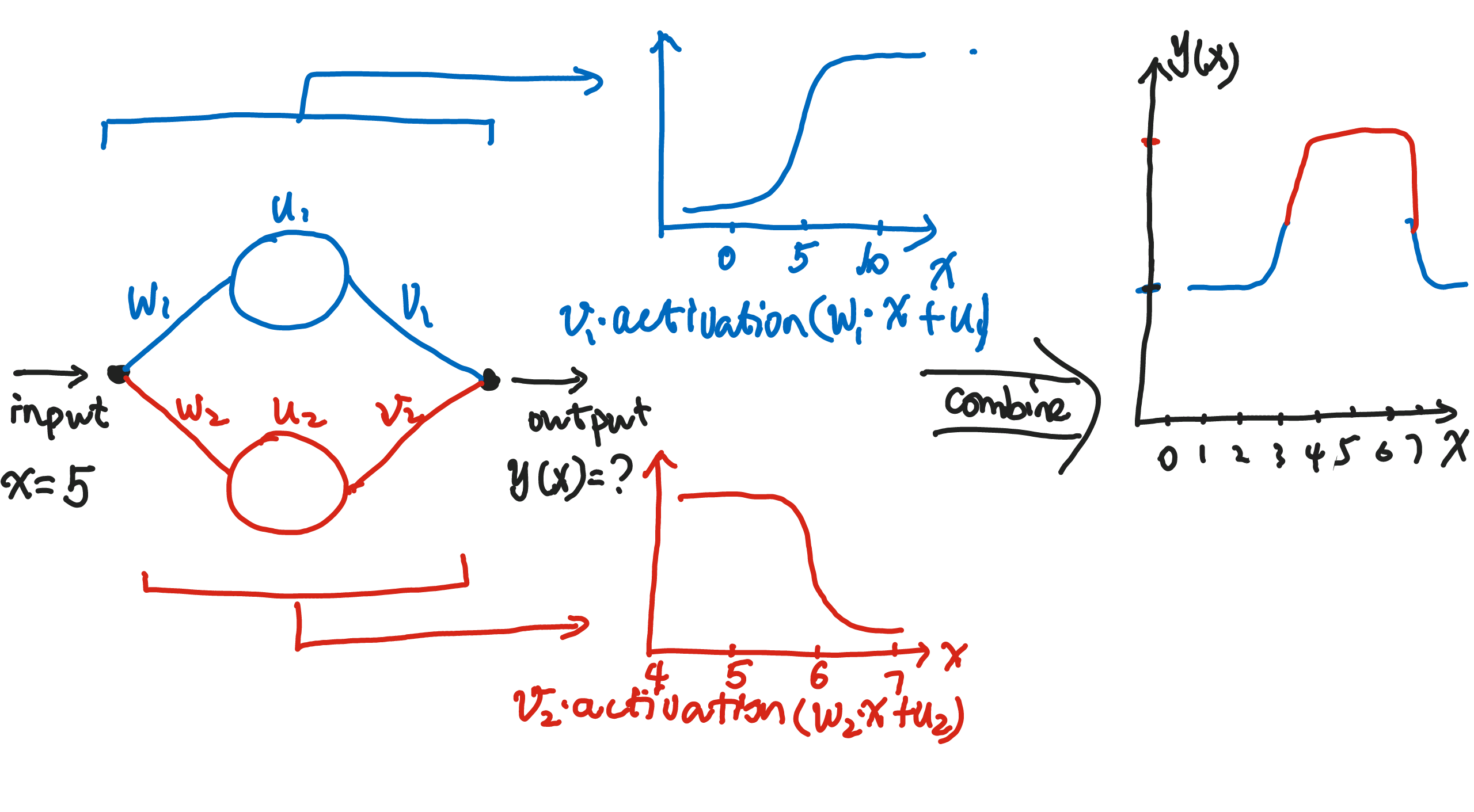Seen from this example, we can expect neural network to be good at classification.

And how is this going to help us with the identification of habitable places? Suppose we have three room with temperature $T_1$, $T_2$, $T_3$ respectively. Only $T_2$ falls into the region of high output value which corresponds to the habitable temperature in our net.

In :
Image(filename='assets/images/2neuronClassification.png',width=900)

Out: